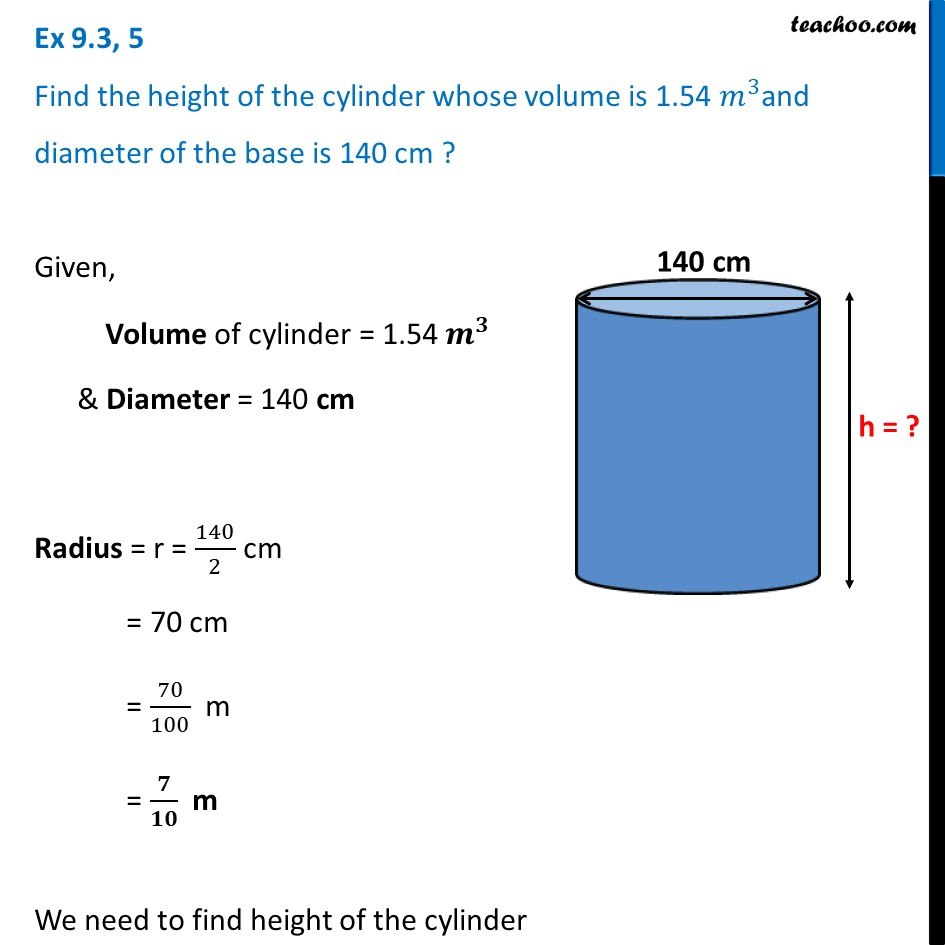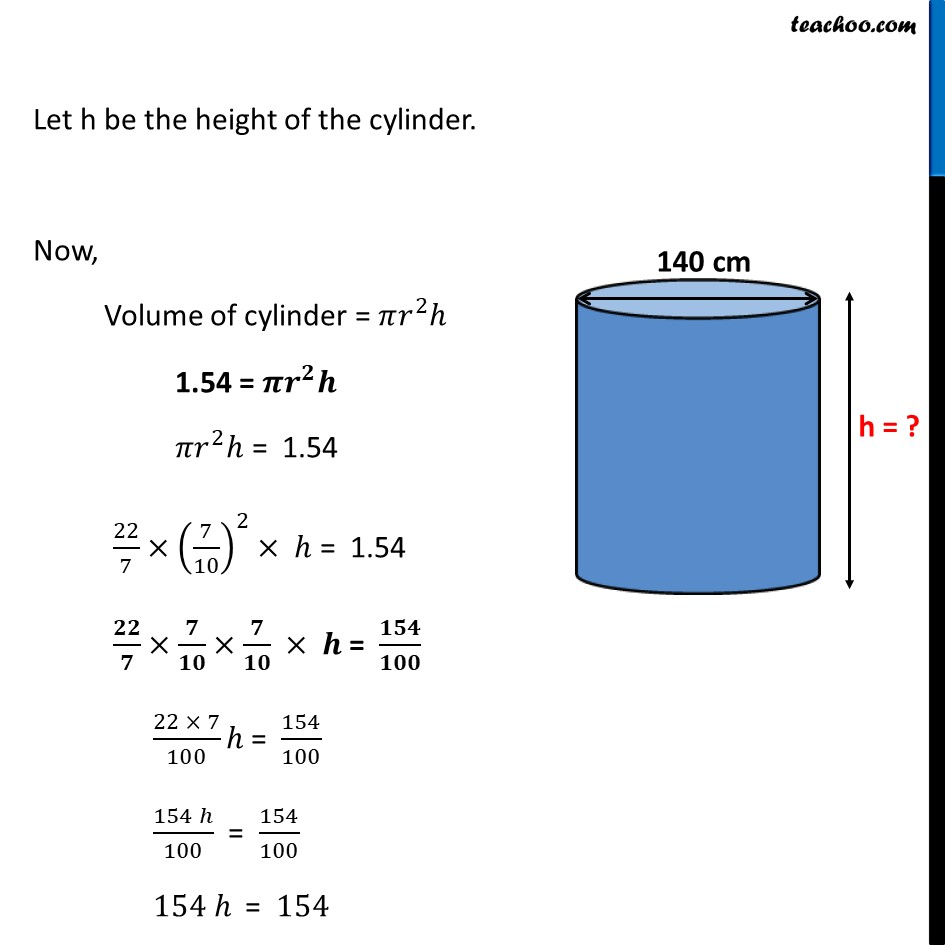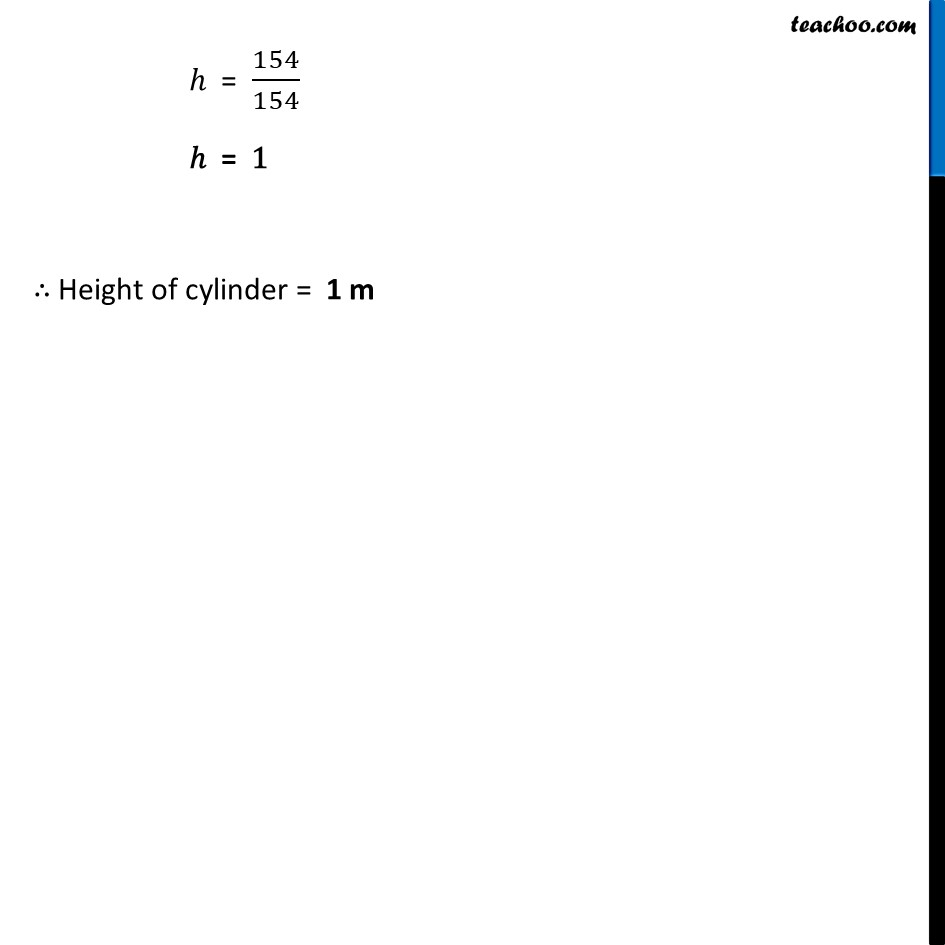Ex 9.3

Chapter 9 Class 8 Mensuration
Serial order wiseLearn in your speed, with individual attention - Teachoo Maths 1-on-1 Class

### Transcript

Ex 9.3, 5 Find the height of the cylinder whose volume is 1.54 𝑚^3and diameter of the base is 140 cm ?Given, Volume of cylinder = 1.54 𝒎^𝟑 & Diameter = 140 cm Radius = r = 140/2 cm = 70 cm = 70/100 m = 𝟕/𝟏𝟎 m We need to find height of the cylinder Let h be the height of the cylinder. Now, Volume of cylinder = 𝜋𝑟^2 ℎ 1.54 = 𝝅𝒓^𝟐 𝒉 𝜋𝑟^2 ℎ = 1.54 22/7×(7/10)^2× ℎ = 1.54 𝟐𝟐/𝟕×𝟕/𝟏𝟎×𝟕/𝟏𝟎 × 𝒉 = 𝟏𝟓𝟒/𝟏𝟎𝟎 (22 × 7)/100 ℎ = 154/100 (154 ℎ)/100 = 154/100 154 ℎ = 154 ℎ = 154/154 ℎ = 1 ∴ Height of cylinder = 1 m